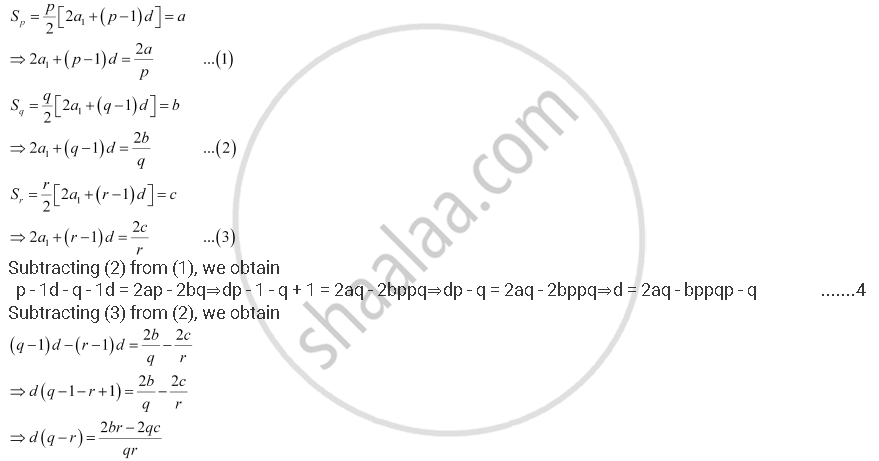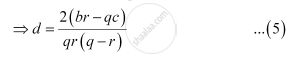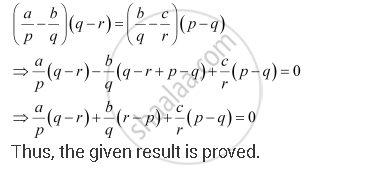CBSE (Arts) Class 11CBSE
Share
Notifications

View all notifications
Books Shortlist
Your shortlist is empty

# Sum of the First P, Q And R Terms of an A.P. Are A, B And C, Respectively. Prove that A/P (Q - R) + B/Q (R- P) + C/R (P - Q) = 0 - CBSE (Arts) Class 11 - Mathematics

Login
Create free account

Forgot password?
ConceptArithmetic Progression (A.P.)

#### Question

Sum of the first p, q and r terms of an A.P. are a, b and c, respectively.

Prove that a/p (q - r) + b/q (r- p) + c/r (p - q) = 0

#### Solution

Let a1 and d be the first term and the common difference of the A.P. respectively.

According to the given informationEquating both the values of d obtained in (4) and (5), we obtain

aq - bppqp - q = br - qcqrq - r⇒aq - bppp - q = br - qcrq - r⇒rq - raq - bp = pp - qbr - qc⇒raq - bpq - r = pbr - qcp - q⇒aqr - bprq - r = bpr - cpqp - q

Dividing both sides by pqr, we obtainIs there an error in this question or solution?

#### APPEARS IN

NCERT Solution for Mathematics Textbook for Class 11 (2018 to Current)
Chapter 9: Sequences and Series
Q: 11 | Page no. 185
Solution Sum of the First P, Q And R Terms of an A.P. Are A, B And C, Respectively. Prove that A/P (Q - R) + B/Q (R- P) + C/R (P - Q) = 0 Concept: Arithmetic Progression (A.P.).
S